Home > Blog

# How to convert an Excel Gantt chart into a MS Project plan

Converting an excel Gantt chart into an MS project plan can be a pain.  It isn’t too bad if the project plan is only a few lines long; however, when the plan is larger (either in terms of the number of tasks or the length of the timescale), it can be time consuming and error-prone.

To make this easier, you can use VBA, and this article will step you through this even if you aren’t familiar with VBA.

For this example, we will be using a very simple Gantt: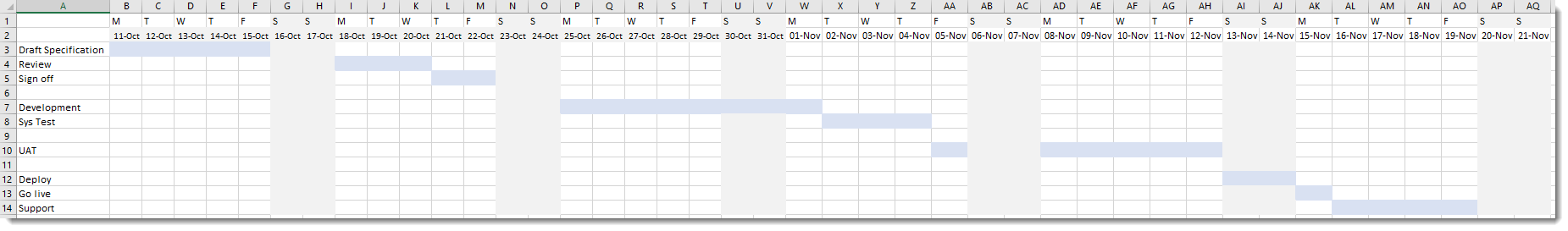## Setup Excel Gantt chart

First, add some columns to the left of the Gantt section where the start and finish will be displayed: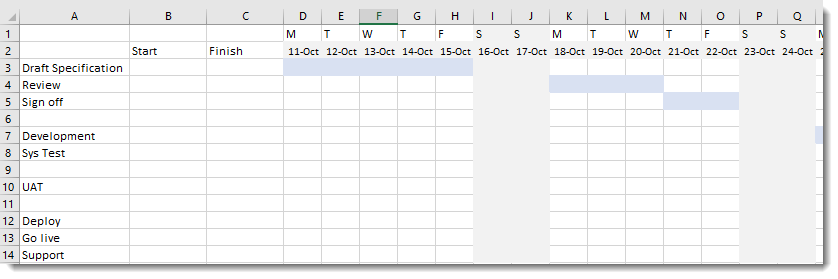## Enter VBA Code

This method can be thought of as reverse Conditional Formatting - the VAB code will look at each cell in the Gantt chart and if it contains a colour the code will display the date for that column. Then it is a simple min / max formula to display the start and finish dates.

Bring up the VBA Window by pressing the <ALT> and <F11> keys.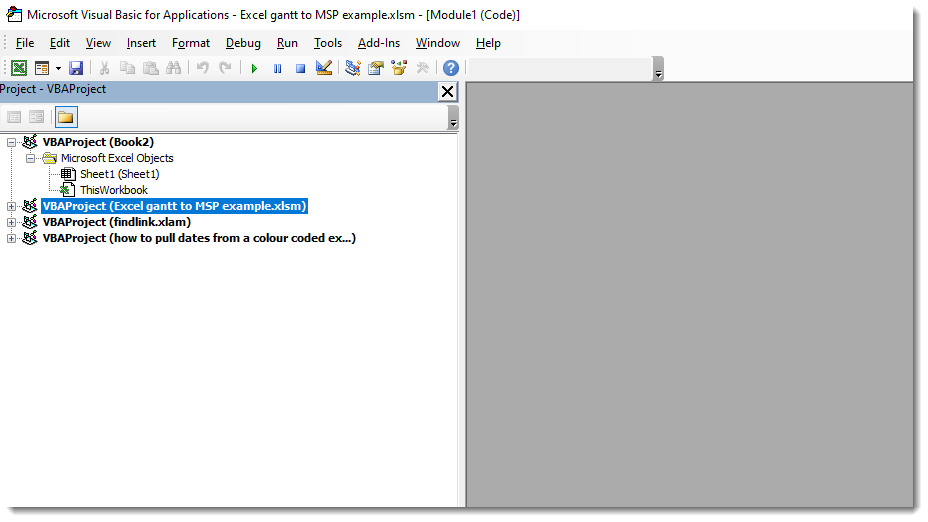Select your file from the list on the left (if it isn’t already selected) and click on the Insert Menu and choose “module”.  Excel will display a blank white space to the right.

Into this area, copy the code below, from Sub to End Sub inclusive.

`Sub Dates_from_colours_not_grey() ' return date values into cell if the cell is not a grey colour (colour index = 2)`

`Dim vlimit As Long`

`Dim hlimit As Long`

`Dim c As Long`

`Dim r As Long`

`On Error GoTo Finalise`

`Application.ScreenUpdating = False`

`Application.Calculation = xlManual`

`Print out the contents of a cell which you want to ignore, count how many Rows and then Coloums the cell is at and enter in r,c format`

`Debug.Print "value = " & Cells(2, 9).Value`

`Debug.Print "colour = " & Cells(2, 9).Interior.ColorIndex`

`vlimit = Cells(Rows.Count, 1).End(xlUp).Row 'count across to the column containing the tasks - tells Excel to count how many rows of data`

`hlimit = Cells(2, Columns.Count).End(xlToLeft).Column ' count down to the Date bar - tells Excel to count how many columns there are`

`For r = 3 To vlimit 'set R to be the first Row which contains data`

`    For c = 4 To hlimit 'set C to be the first column that contains dates`

`       If Cells(r, c).Interior.ColorIndex <> 2 And Cells(r, c).Interior.ColorIndex <> -4142 Then`

`           Cells(r, c) = Cells(2, c).Value 'the "2" number is the row which contains the date information`

`           Else: Cells(r, c) = ""`

`       End If`

`    Next c`

`Next r`

`MsgBox "Complete"`

`Finalise:`

`Application.ScreenUpdating = True`

`Application.Calculation = xlAutomatic`

`End Sub`

This code is set up for the example shown at the top of this article.  As such, you will likely need to change some of the variables in it.  This code using the R1C1 type reference system rather than the A1 that you will be familiar with from entering a formula in a cell.  Thus you will need to count rows and columns rather than using letters and numbers.

• vlimit = Cells(Rows.Count, 1).End(xlUp).Row– Change the 1 to the column number which contains the tasks in your Excel Gantt
• hlimit = Cells(2, Columns.Count).End(xlToLeft).Column– change the 2 to the row number which contains your timescale across the plan
• For r = 3 To vlimit - Change the 3 to the row number of your first task
• For c = 4 To hlimit - Change the 4 to the column number which contains your first date
• Cells(r, c) = Cells(2, c).Value - change the 2 to the row number which contains your timescale across the plan

If you don’t know the cell colour used for dates you want to ignore (in this example, the shading for weekends), you can find this out by changing the row and column numbers in the code

Debug.Print "value = " & Cells(2, 9).Value the 2 and 9 for the row and column, respectively, for a cell with the same shading.  In the example, this is looking at cell I2.

You will need to be able to see the “immediate” window in the VBA display.  If this isn’t showing, click on the “view” menu and chose “immediate window”.  Then click on the line which contains “vlimit = ….” and click on the “debug” menu and select “run to cursor”.  The colour index and contents of the cell will now be “printed” into the immediate window.  Now select the reset button to prevent the rest of the code from running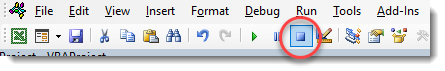Once you have this, you can change the “2” entry in the code below to the correct colour index value

“If Cells(r, c).Interior.ColorIndex <> 2 And Cells(r, c).Interior.ColorIndex <> -4142 Then”

Get the start and finish dates

Now your code is all complete, and you can run it.  To do this, click back into your Gantt chart sheet to ensure that it is the active sheet.  Then click into the VBA code you edited.

Click on the run button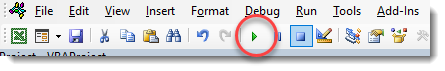Once the code has finished running, it will display the message “complete”, and each of the shaded cells will be showing the corresponding date from your timescale row.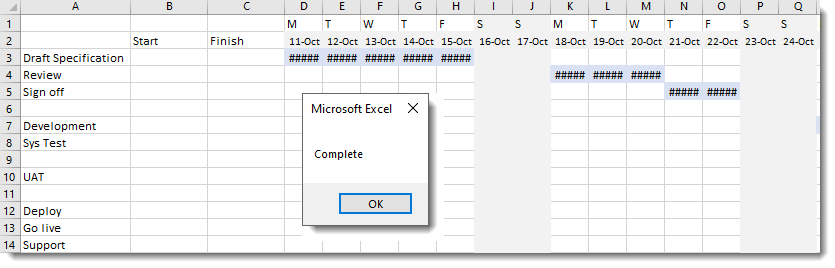Now it is a simple matter of displaying the minimum date on each row as the Start Date and the maximum date as the finish date.  To do this in the example, you would enter

B3 =IF(MIN(D3:AS3)=0,"",MIN(D3:AS3))

C3 =IF(B3="","",MAX(D3:AS3))

Then copy these down to cover all the rows of tasks: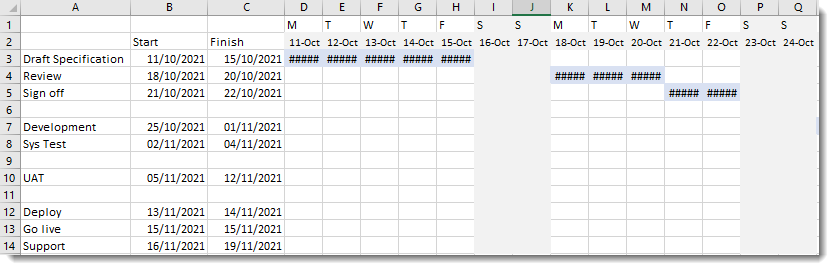## Copy into MS Project

To make the required MS Project plan, all you have to do is copy the task rows into the MS Project plan’s “name” column and the Start and Finish columns into the respective Start and Finish columns: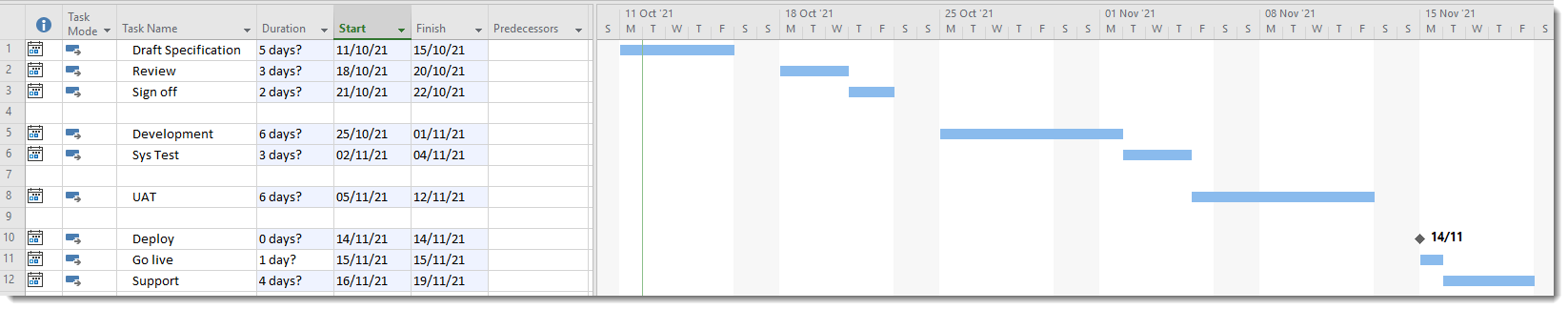It is good practice to remove blank rows and then link tasks related to each other and add summary rows where sensible.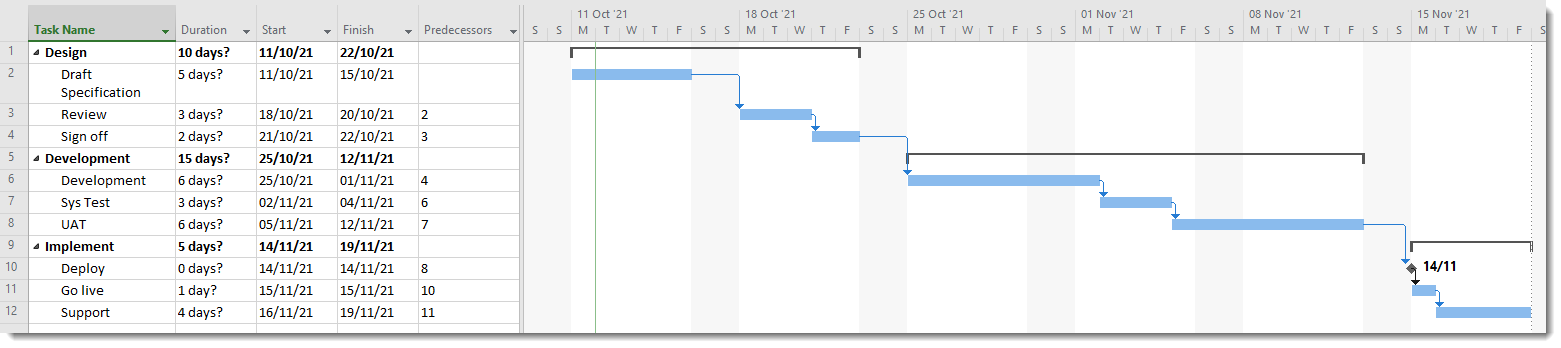## Variations in the Excel Gantt – what if it isn’t in days?

Note that if your timescale is in weeks, you will need to change the Finish formula to display the Friday of each week

C3 =IF(B3="","",MAX(D3:AS3)+5)

If your timescale is in Months, you will need to change the timescale to show the 1st of each month.  This means that the macro displays date rather than text so that the min and max formulas can find the start and finish.

To do this, enter the 1st of the initial month (for instance, replace Jan with 1/1/21).  In the cell next to it (in the example D2) enter the formula

D2=DATE(YEAR(D2),MONTH(D2)+1,1)

Copy this across the timescale.

The Finish calculation will now be:

C3 =IF(B3="","",EOMONTH(MAX(D3:AS3),0))Miles Goodchild has been a Program and Programme Planner, PMO lead and Project Manger since 1998.  He enjoys using MS Office, especially Project, Excel and Visio to make life easier and simpler.  In the course of this he created SummaryPro.  In this blog he shares some of the tips and techniques he has learnt over the years in the hope that they will be useful to you.